# Cash Flow From Operations Ratio## What is Cash Flow from Operations Ratio?

The term “cash flow from operations ratio” refers to the coverage Ratio that assesses how adequately the Cash Flow generated from the operation will be able to pay off the current liabilities of the company. In other words, it is the liquidity ratio that measures a company’s ability to cover its short term financial obligations with its operating cash flow or it is earning from operations per dollar of current liability. This ratio can be very helpful if used in conjunction with other liquidity ratios like cash ratio, quick ratio, current ratio, etc.

Formula

The formula for CFO Ratio can be derived by dividing the cash flow from operating activities by the total current liabilities. Mathematically, it is represented as,

Cash Flow from Operations Ratio = Cash Flow from Operations / Current Liabilities

The cash flow from operations is either easily available from the cash flow statement or can be computed by adding net income, non-cash charges, and change in working capital, while current liabilities include trade payables, accrued expense, current portion of long term debt, short term borrowing, etc.

Cash Flow from Operations = Net Income + Non-Cash Charges (Like Depreciation & Amortization Expense) + Changes in Working Capital

### Examples of Cash Flow From Operations Ratio (With Excel Template)

Let’s take an example to understand the calculation of the CFO Ratio formula in a better manner.

You can download this Cash Flow from Operations Ratio Excel Template here – Cash Flow from Operations Ratio Excel Template

#### Example – #1

Let us take the example of company JKL Inc. which is in the business of wholesale furniture trading in California. Recently the company has published its annual report and the following financial information has been made available: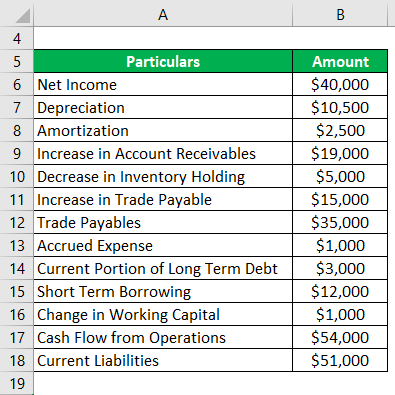Solution:

Change in Working Capital is calculated as,

Change in Working Capital = ( – Increase in Account Receivables + Decrease in Inventory Holding + Increase in Trade Payable)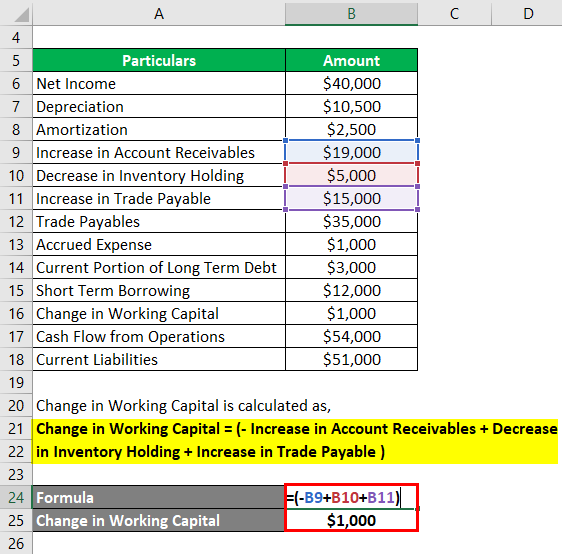• Change in Working Capital = – \$19,000 + \$5,000 + \$15,000
• Change in Working Capital = \$1,000

Cash Flow from Operations is calculated as,

Cash Flow from Operations = Net Income + Depreciation + Amortization + Change in Working Capital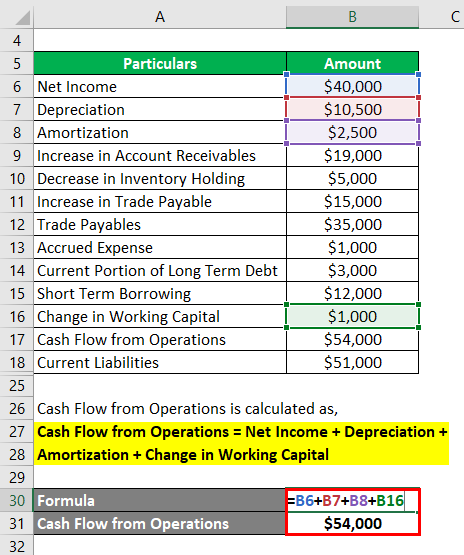• CFO = \$40,000 + \$10,500 + \$2,500 + \$1,000
• CFO = \$54,000

Current Liabilities is calculated as,

Current Liabilities = Trade Payables + Accrued Expense + Current Portion of Long Term Debt + Short Term Borrowing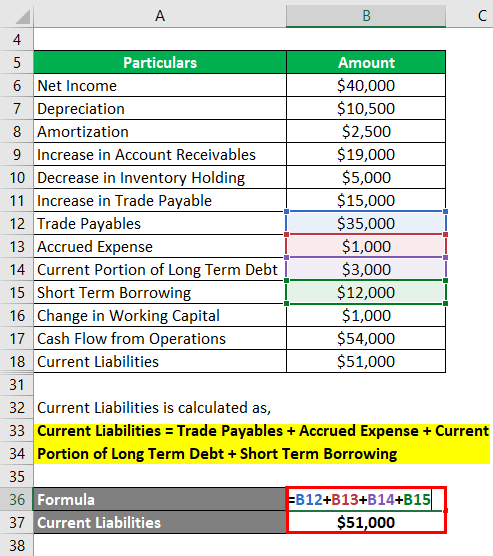• Current Liabilities = \$35,000 + \$1,000 + \$3,000 + \$12,000
• Current Liabilities = \$51,000

CFO Ratio is calculated by using the formula given below

Cash Flow from Operations Ratio = Cash Flow from Operations / Current Liabilities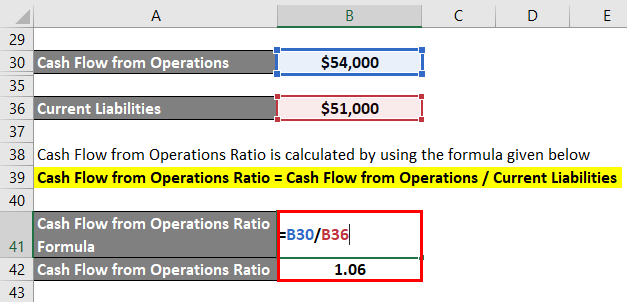• CFO Ratio = \$54,000 / \$51,000
• CFO Ratio = 1.06

Therefore, JKL Ltd has a cash flow ratio of 1.06 which indicates that the company earns S1.06 from operating activities for every dollar of current liabilities.

#### Example – #2

Let us take the example of Apple Inc. to calculate the cash flow from operations ratio based on the annual report for the year ended on September 29, 2018. The following information is available: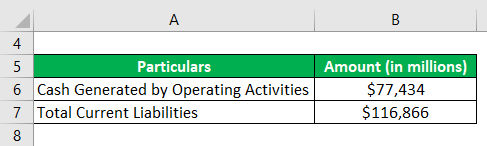Solution:

CFO Ratio is calculated by using the formula given below

Cash Flow from Operations Ratio = Cash Flow from Operations / Current Liabilities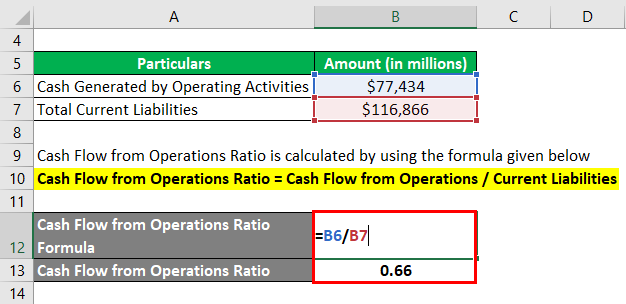• CFO Ratio = \$77,434 Mn / \$116,866 Mn
• CFO Ratio = 0.66

Therefore, Apple Inc. had a cash flow ratio of 0.66 which indicates that it can cover up to 66% of the current liabilities with its cash flow from operating activities.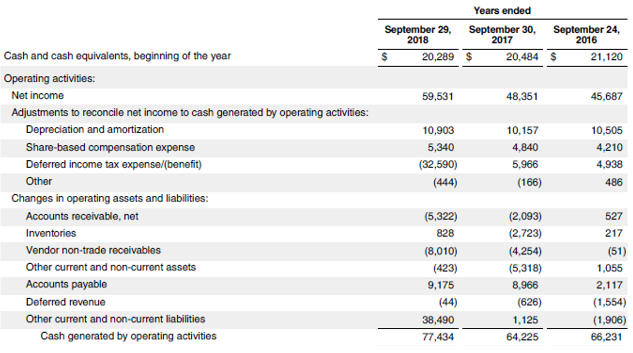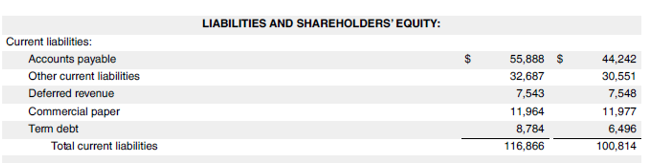Source: d18rn0p25nwr6d.cloudfront.net

#### Example – #3

Let us take the example of Walmart which is a multinational supermarket chain. Calculate the cash flow from operations ratio for Walmart based on the financial information from the annual report for 2019: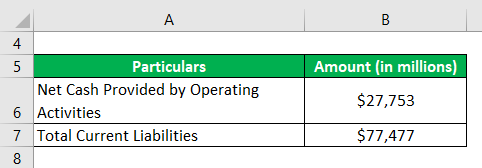Solution:

CFO Ratio is calculated by using the formula given below

Cash Flow from Operations Ratio = Cash Flow from Operations / Current Liabilities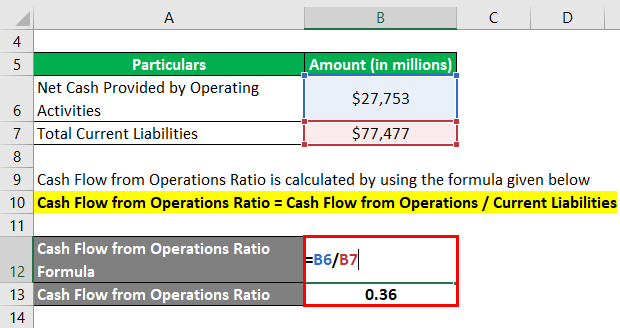• CFO Ratio = \$27,753 Mn / \$77,477 Mn
• CFO Ratio = 0.36

Therefore, Walmart had a cash flow ratio of 0.36 which indicates that it can cover up to 36% of the current liabilities with its cash flow from operating activities.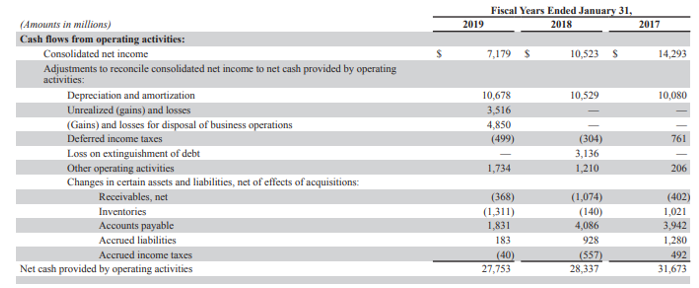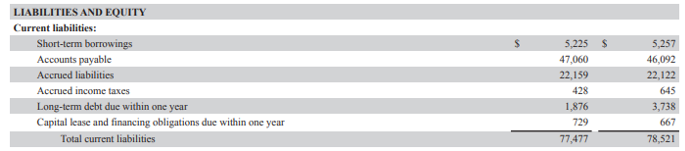Source: https://s2.q4cdn.com/056532643

Some of the advantages and disadvantages of CFO Ratio are as follows:

The advantage of CFO Ratio is that it is much better than most other liquidity ratios because it assesses the liquidity coverage on the basis of cash generated from the core business operation.

The disadvantage of the CFO Ratio is that this ratio is vulnerable to manipulation by companies. However, the manipulations are not as easy as it is in the case of net income.

### Conclusion

So, this is another liquidity metric that measures how well the operating cash flow of a company can cover its current liabilities. Higher the value of the ratio, the better is the liquidity position of the company.

### Recommended Articles

This is a guide to the Cash Flow From Operations Ratio. Here we discuss the introduction, top 3 examples, advantages, and disadvantages along with downloadable excel template. You can also go through our other suggested articles to learn more –

1. Elasticity of Demand Example
2. Economies of Scale Example
3. Cash Reserve Ratio
4. Cash Ratio

The post Cash Flow From Operations Ratio appeared first on EDUCBA.

This post first appeared on Free Online CFA Calculator Training Course | EduCB, please read the originial post: here